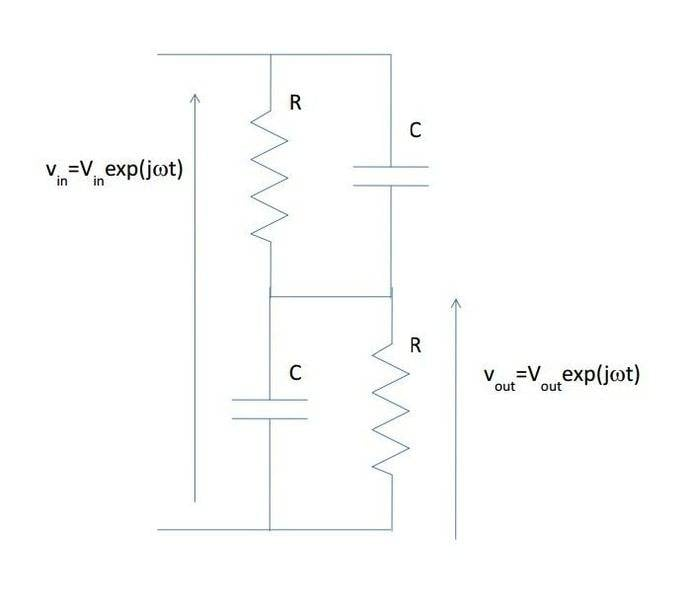# How to find the impedance on this circuit

• Engineering

## Homework Statement

[/B]
I need to find the total impedance for this circuit below. No values are given so I just need to write out an equation.## The Attempt at a Solution

I'm having a hard time deciding what is in parallel/series. I took a guess and came up with:

( R * 1/jwc / R + 1/jwc ) + ( R * 1/jwc / R + 1/jwc )

So I assumed the top two components are in parallel, same with the bottom two.

NascentOxygen
Staff Emeritus
I'm having a hard time deciding what is in parallel/series. I took a guess and came up with:

( R * 1/jwc / R + 1/jwc ) + ( R * 1/jwc / R + 1/jwc )
Parentheses are free, so use all that are needed. This expression is incomplete; it might mean something to you, to everyone else we can only guess. Except for some notable exceptions, whitespace means nothing in mathematical expressions.

So I assumed the top two components are in parallel, same with the bottom two.
Yes, and those pairs are themselves connected in series.

If the R's are equal, likewise the C's, then you could find total Z by just doubling the impedance of one RC combo.

•Arabcha
The question is not clear.
Do you speak about the INPUT impedance as seen by Vin or the OUTPUT impedance as seen by Vout?
(By the way: From your answer I can imagine what you mean - nevertheless, you should learn to ask clear questions).